Question

# UPDATE THE UNIVERSE PROGRAM: C. Research the size of each CelestialObject and its distance from the...

UPDATE THE UNIVERSE PROGRAM:

C. Research the size of each CelestialObject and its distance from the sun (for planets) and the distance of the moons from the planets
D. Calculate the distance between any two CelestialObject (e.g. between 2 planets and between two moons)

COMPLETE STEPS C AND D and THE MODIFICATION BELOW.

1. GALAXY CLASS

//**MODIFICATION NEEDED**
//Use the DBPM format to complete the class.
//Review the SolarSystem class for the Star attribute as an example to follow.
//For the solarSystem attribute:
// Create 1 default constructor, 1 overloaded constuctor, 1 setter, 1 getter
class Galaxy {
SolarSystem solarSystem;
}

2. UNIVERSE CLASS

//**MODIFICATION NEEDED**
//Use the DBPM format to complete the class.
//Review the SolarSystem class for the Star attribute as an example to follow.
//Create a galaxy attribute of type Galaxy:
//Create 1 default constructor, 1 overloaded constuctor, 1 setter, 1 getter
public class Universe {
// Create a galaxy attritute of type Galaxy

......

}

3. MAIN FUNCTION

// Create a solarSystem called InterStellerSpace
// Set Sun (which is a star) to the SolarSystem InterStellerSpace
// Set the Planets, Earth and Mars to the SolarSystem InterStellerSpace
// Create a galaxy object called MilkyWay
// Set InterStellerSpace solarSystem to the galaxy MilkyWay

---------------------------------------------------------------------------------------

/*

Modify the existing program to expand the current universe.

A. Add one Star called Sun

B. Two planets called Earth (name="Blue Planet") with one moon (called blueMoon),

and Mars (name="Red Planet") with two moons (Deimus and Phobos).

C. Research the size of each CelestialObject and its distance from the sun (for

planets) and the distance of the moons from the planets

D. Calculate the distance between any two CelestialObject (e.g. between 2 planets

and or between two moons

1. Follow the naming patterns used by DBPM for all classes

required

*/

package universe;

import java.util.ArrayList;

// NO CHANGES REQUIRED FOR THIS CLASS

// CelestialObject is a fully DBPM implemented class with an abstract method:

// a default constructor, overloaded constructors, setters and getters

abstract class CelestialObject {

private String name; // name attribute

private double size; // size attribute

private long distance; // distance attribute

public CelestialObject() { this.name = ""; this.size =

0.0; this.distance = 0; } // default constructor

public CelestialObject(String name) { this.name = name; this.size =0.0;

this.distance = 0;

}

public CelestialObject(double size) { this.name = ""; this.size =size;this.distance = 0; }

public CelestialObject(int distance) { this.name = ""; this.size =0.0; this.distance = distance; }

public CelestialObject(String name,

double size,

int distance) { this.name = name; this.size =

size; this.distance = distance; }

public void setName(String name) { this.name = name; }

//setter method name

public void setSize(double size) { this.size = size; }

//setter method size

public void setDistance(long distance){ this.distance = distance; }

//setter method distance

public String getName() { return this.name; } //getter method name

public double getSize() { return this.size; } //getter method size

public long getDistance() { return this.distance; } //getter method distance

abstract public double calculateDistanceBetweenCelestialObject(CelestialObject otherObj);//abstract method

}

class Star extends CelestialObject {

public Star()

{

super();

}

public Star(String name, double size)

{

setName(name);

setSize(size);

}

@Override

public double calculateDistanceBetweenCelestialObject(CelestialObject otherObj) {

// TODO Auto-generated method stub

return this.getDistance() - otherObj.getDistance();

} // Star Class

}

class Moon extends CelestialObject {

public Moon()

{

super();

}

public Moon(String name, double size, long distance)

{

setName(name);

setSize(size);

setDistance(distance);

}

@Override

public double calculateDistanceBetweenCelestialObject(

CelestialObject otherObj) {

return this.getDistance() - otherObj.getDistance();

} // Moon Class

}

class Planet extends CelestialObject { // Planet Class

private ArrayList<Moon> moon = new ArrayList<Moon> () ;

// moon attribute

public Planet() {} // default constructor

public Planet(Moon moon)

{

} // adding a moon the the planet

public Planet(ArrayList moon)

{ this.moon = moon; } // adding an array of moons the the planet

public void setMoon(Moon moon) { this.moon.add(moon); } // adding a moon the the planet

public void setMoon(ArrayList moon)

{ this.moon = moon; } // adding an array of moons the the planet

public ArrayList getMoon() { return this.moon; } // get the array of moons

public Moon getMoon(int position)

{

return this.moon.get(position); }

// get an individual moon

public double calculateDistanceBetweenCelestialObject(CelestialObject

otherPlanet) {

return this.getDistance() - otherPlanet.getDistance();}

}

class SolarSystem {

private Star star;

private ArrayList<Planet> planet = new ArrayList<Planet> () ;

public SolarSystem() {} // default constructor

public SolarSystem(Star star) { this.star = star; } // overloaded constructor star

public SolarSystem(Star star, Planet planet)

{ this.star = star;

public SolarSystem(Star star, ArrayList planet)

{ this.star = star; this.planet =

planet; } // adding a star/planets to the solarsystem

public void setStar(Star star) { this.star = star; } // setter method name

public Star getStar() { return this.star; } // getter method name

public void setPlanet(Planet planet)

{

}

public void setPlanet(ArrayList planet)

{

this.planet = planet;

}

public ArrayList getPlanet()

{

return this.planet;

}

public Planet getPlanet(int position)

{

return this.planet.get(position);

}

}

class Galaxy {

SolarSystem solarSystem;

public Galaxy()

{

}

public Galaxy(SolarSystem ss)

{

this.solarSystem = ss;

}

public void setSolarSystem(SolarSystem ss)

{

solarSystem = ss;

}

public SolarSystem getSolarSystem()

{

return solarSystem;

}

}

public class Universe {

// Create a galaxy attritute of type Galaxy

//**MODIFICATION NEEDED**

public static void main(String[] args) {

// Create a star call Sun and set the name to SOL, set the size, no

//distance is needed

Star sun = new Star("SOL", 864938.0);

// Create two planet objects called Earth and Mars

// Set the Planet names (Blue Planet and Red Planet), the size of

//each planet and the distance from the sun

Planet Earth = new Planet();

Planet Mars = new Planet();

Earth.setName("Blue Planet");

Earth. setSize(3959.0);

Earth.setDistance(92960000);

Mars.setName("Red Planet");

Mars.setSize(2106.0);

Mars.setDistance(141600000);

// Create one moon for Earth called BlueMoon (named Blue moon), set

//the size and the distance from the Earth object

Moon BlueMoon = new Moon();

BlueMoon.setName("Blue Moon");

BlueMoon.setSize(1097.6);

BlueMoon.setDistance(238900);

// set the BlueMoon to Earth object

Earth.setMoon(BlueMoon);

// Create two moons for Mars called Phobos and Deimus (use as the

//name), set the size and the distance from the Mars object

Moon Phobos = new Moon();

Phobos.setName("Phobos");

Phobos.setSize(6.9);

Phobos.setDistance(5738);

Moon Deimus = new Moon();

Deimus.setName("Deimus");

Deimus.setSize(3.9);

Deimus.setDistance(14576);

// set the two moons to Mars

Mars.setMoon(Phobos);

Mars.setMoon(Deimus);

// Create a solarSystem called InterStellerSpace

SolarSystem InterStellerSpace = new SolarSystem();

// Set Sun (which is a star) to the SolarSystem InterStellerSpace

InterStellerSpace.setStar(sun);

// Set the Planets, Earth and Mars to the SolarSystem

//InterStellerSpace

InterStellerSpace.setPlanet(Earth);

InterStellerSpace.setPlanet(Mars);

// Create a galaxy object called MilkyWay

Galaxy MilkyWay = new Galaxy();

// Set InterStellerSpace solarSystem to the galaxy MilkyWay

MilkyWay.setSolarSystem(InterStellerSpace);

// NO CHANGES REQUIRED

// This will test your logic and should show the sun, two planets

//with their own moons and all the details

System.out.println("The Solor System contains the following");

System.out.println("-- Sun Information --");

System.out.println(" Name : " + MilkyWay.getSolarSystem().getStar().getName());

System.out.println(" Size : " + MilkyWay.getSolarSystem().getStar().getSize());

for (int i = 0; i < MilkyWay.getSolarSystem().getPlanet().size();

i ++){

System.out.println(" ++ Planet Information ++");

System.out.println(" Name : " + MilkyWay.getSolarSystem().getPlanet(i).getName());

System.out.println(" Size : " + MilkyWay.getSolarSystem().getPlanet(i).getSize());

System.out.println(" Distance: " + MilkyWay.getSolarSystem().getPlanet(i).getDistance());

// for the moons for each planet

for (int m = 0; m < MilkyWay.getSolarSystem().getPlanet(i).getMoon().size(); m ++){

System.out.println(" !! Moon Information !!");

System.out.println(" Name : " + MilkyWay.getSolarSystem().getPlanet(i).getMoon(m).getName());

System.out.println(" Size : " + MilkyWay.getSolarSystem().getPlanet(i).getMoon(m).getSize());

System.out.println(" Distance: " + MilkyWay.getSolarSystem().getPlanet(i).getMoon(m).getDistance());

}

}

//**MODIFICATION NEEDED**

// Calculate the distance between the two planets

System.out.println(" The Distance between Earth Mars is " + Earth.calculateDistanceBetweenCelestialObject(Mars));

}

}

//CelestialObject.java

package com.universe;

//NO CHANGES REQUIRED FOR THIS CLASS

//CelestialObject is a fully DBPM implemented class with an abstract method:

//a default constructor, overloaded constructors, setters and getters

abstract class CelestialObject {

private String name; // name attribute

private double size; // size attribute

private long distance; // distance attribute

public CelestialObject() {
this.name = "";
this.size = 0.0;
this.distance = 0;
} // default constructor

public CelestialObject(String name) {
this.name = name;
this.size = 0.0;
this.distance = 0;
}

public CelestialObject(double size) {
this.name = "";
this.size = size;
this.distance = 0;
}

public CelestialObject(int distance) {
this.name = "";
this.size = 0.0;
this.distance = distance;
}

public CelestialObject(String name, double size, int distance) {
this.name = name;
this.size = size;
this.distance = distance;
}

public void setName(String name) {
this.name = name;
}

// setter method name

public void setSize(double size) {
this.size = size;
}

// setter method size

public void setDistance(long distance) {
this.distance = distance;
}

// setter method distance

public String getName() {
return this.name;
} // getter method name

public double getSize() {
return this.size;
} // getter method size

public long getDistance() {
return this.distance;
} // getter method distance

abstract public double calculateDistanceBetweenCelestialObject(CelestialObject otherObj);// abstract method

}

//Star.java

package com.universe;

class Star extends CelestialObject {

public Star()
{
super();
}

public Star(String name, double size)
{
setName(name);
setSize(size);
}

@Override
public double calculateDistanceBetweenCelestialObject(CelestialObject otherObj) {

// TODO Auto-generated method stub

return this.getDistance() - otherObj.getDistance();

} // Star Class

}

//Moon.java

package com.universe;

class Moon extends CelestialObject {

public Moon()
{
super();
}

public Moon(String name, double size, long distance)
{
setName(name);
setSize(size);
setDistance(distance);
}

@Override
public double calculateDistanceBetweenCelestialObject(CelestialObject otherObj) {

return this.getDistance() - otherObj.getDistance();

} // Moon Class

}

//Planet.java

package com.universe;

import java.util.ArrayList;

class Planet extends CelestialObject { // Planet Class

private ArrayList<Moon> moon = new ArrayList<Moon>();

// moon attribute

public Planet() {
} // default constructor

public Planet(Moon moon)

{

} // adding a moon the the planet

public Planet(ArrayList<Moon> moon)
{
this.moon = moon;
} // adding an array of moons the the planet

public void setMoon(Moon moon) {
} // adding a moon the the planet

public void setMoon(ArrayList<Moon> moon)
{
this.moon = moon;
} // adding an array of moons the the planet

public ArrayList<Moon> getMoon() {
return this.moon;
} // get the array of moons

public Moon getMoon(int position)
{
return this.moon.get(position);
}

// get an individual moon

public double calculateDistanceBetweenCelestialObject(CelestialObject otherPlanet) {

return this.getDistance() - otherPlanet.getDistance();
}

}

//SOlarSystem.java

package com.universe;

import java.util.ArrayList;

class SolarSystem {

private Star star;

private ArrayList<Planet> planet = new ArrayList<Planet>();

public SolarSystem() {
} // default constructor

public SolarSystem(Star star) {
this.star = star;

public SolarSystem(Star star, Planet planet)

{
this.star = star;

} // adding a star/planet to the solarsystem

public SolarSystem(Star star, ArrayList planet)

{
this.star = star;
this.planet =

planet;
} // adding a star/planets to the solarsystem

public void setStar(Star star) {
this.star = star;
} // setter method name

public Star getStar() {
return this.star;
} // getter method name

public void setPlanet(Planet planet)

{

}

public void setPlanet(ArrayList planet)

{

this.planet = planet;

}

public ArrayList getPlanet()

{

return this.planet;

}

public Planet getPlanet(int position)

{

return this.planet.get(position);

}

}

//Galaxy.java

package com.universe;

class Galaxy {

SolarSystem solarSystem;

public Galaxy()
{

}

public Galaxy(SolarSystem ss)
{
this.solarSystem = ss;
}

public void setSolarSystem(SolarSystem ss)
{
solarSystem = ss;
}

public SolarSystem getSolarSystem()
{
return solarSystem;
}

}

//Universe.java

package com.universe;

public class Universe {

// Create a galaxy attritute of type Galaxy

Galaxy galaxy;

public Universe() {

}

public Universe(Galaxy g) {
this.galaxy = g;
}

public void setGalaxy(Galaxy g) {
galaxy = g;
}

public Galaxy getGalaxy() {
return galaxy;
}

public static void main(String[] args) {

// Create a star call Sun and set the name to SOL, set the size, no distance is needed

Star sun = new Star("SUN", 864938.0);

// Create two planet objects called Earth and Mars

// Set the Planet names (Blue Planet and Red Planet), the size of each planet and the distance from the sun

Planet Earth = new Planet();
Earth.setName("Blue Planet");
Earth.setSize(3959.0);
Earth.setDistance(92960000);

Planet Mars = new Planet();
Mars.setName("Red Planet");
Mars.setSize(2106.0);
Mars.setDistance(141600000);

// Create one moon for Earth called BlueMoon (named Blue moon), set

// the size and the distance from the Earth object

Moon BlueMoon = new Moon();
BlueMoon.setName("Blue Moon");
BlueMoon.setSize(1097.6);
BlueMoon.setDistance(238900);
// set the BlueMoon to Earth object
Earth.setMoon(BlueMoon);

// Create two moons for Mars called Phobos and Deimus (use as the name), set the size and the distance from the Mars object

Moon Phobos = new Moon();
Phobos.setName("Phobos");
Phobos.setSize(6.9);
Phobos.setDistance(5738);

Moon Deimus = new Moon();
Deimus.setName("Deimus");
Deimus.setSize(3.9);
Deimus.setDistance(14576);

// set the two moons to Mars
Mars.setMoon(Phobos);
Mars.setMoon(Deimus);

// Create a solarSystem called InterStellerSpace
SolarSystem InterStellerSpace = new SolarSystem();

// Set Sun (which is a star) to the SolarSystem InterStellerSpace
InterStellerSpace.setStar(sun);

// Set the Planets, Earth and Mars to the SolarSystem InterStellerSpace

InterStellerSpace.setPlanet(Earth);
InterStellerSpace.setPlanet(Mars);

// Create a galaxy object called MilkyWay
Galaxy MilkyWay = new Galaxy();

// Set InterStellerSpace solarSystem to the galaxy MilkyWay
MilkyWay.setSolarSystem(InterStellerSpace);

// NO CHANGES REQUIRED

// This will test your logic and should show the sun, two planets with their own moons and all the details

System.out.println("The Solor System contains the following");
System.out.println("-- Sun Information --");
System.out.println(" Name : " + MilkyWay.getSolarSystem().getStar().getName());
System.out.println(" Size : " + MilkyWay.getSolarSystem().getStar().getSize());
for (int i = 0; i < MilkyWay.getSolarSystem().getPlanet().size(); i++) {
System.out.println(" ++ Planet Information ++");
System.out.println(" Name : " + MilkyWay.getSolarSystem().getPlanet(i).getName());
System.out.println(" Size : " + MilkyWay.getSolarSystem().getPlanet(i).getSize());
System.out.println(" Distance: " + MilkyWay.getSolarSystem().getPlanet(i).getDistance());
// for the moons for each planet
for (int m = 0; m < MilkyWay.getSolarSystem().getPlanet(i).getMoon().size(); m++) {
System.out.println(" !! Moon Information !!");
System.out.println(" Name : " + MilkyWay.getSolarSystem().getPlanet(i).getMoon(m).getName());
System.out.println(" Size : " + MilkyWay.getSolarSystem().getPlanet(i).getMoon(m).getSize());
System.out.println(" Distance: " + MilkyWay.getSolarSystem().getPlanet(i).getMoon(m).getDistance());
}
}

// **MODIFICATION NEEDED**

// Calculate the distance between the two planets

long bluePlanet = Earth.getDistance();
long redPlanet = Mars.getDistance();
System.out.println(" The Distance between Earth and Mars is " + Math.abs(bluePlanet - redPlanet));

long blueMoon = BlueMoon.getDistance();
long phobos = Phobos.getDistance();
System.out.println("The Distance between Blue Moon and Phobos is " + Math.abs(blueMoon - phobos));

long deimus = Deimus.getDistance();
System.out.println("The Distance between Phobos and Deimus is " + Math.abs(phobos - deimus));

}

}

//Output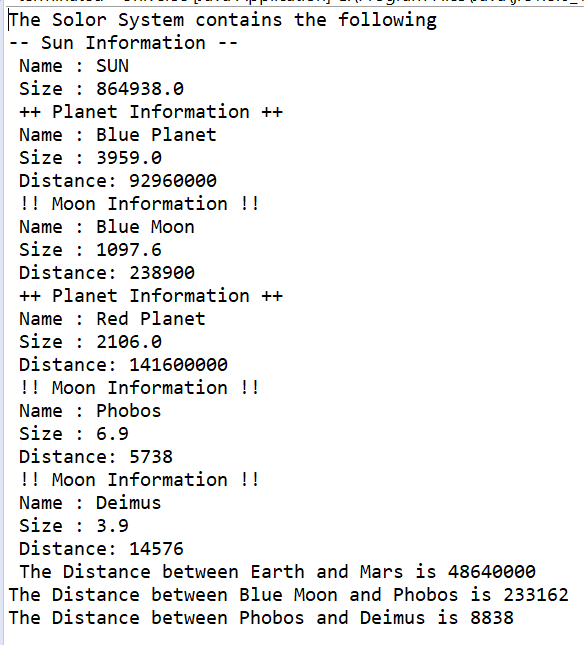#### Earn Coins

Coins can be redeemed for fabulous gifts.

Similar Homework Help Questions
• ### Update your Assignment 4 as follows (Assignment 4 codes will be on the bottom) Create a...

Update your Assignment 4 as follows (Assignment 4 codes will be on the bottom) Create a new method called displayAll, that takes an ArrayList (of your base class) as a parameter, and doesn't return anything [25 pts] The displayAll method will loop through the ArrayList, and call the display (or toString) method on each object   [25 pts] In the main method, create an ArrayList containing objects of both your base class and subclass. (You should have at least 4 objects)  [25...

• ### So i need help with this program, This program is supposed to ask the user to...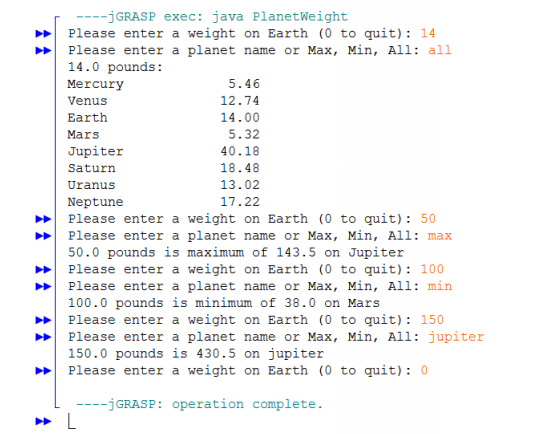So i need help with this program, This program is supposed to ask the user to input their weight on earth so I would put "140" then it would ask "enter planet name, Min, Max or all" and if the user typed in all it would display all the planets and your weight on them. Right now Im suck on the part where im required to do a switch statement with a linear search. Below this im going to put...

• ### How to solve my code for my Deliv C program?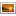ProgramIntro.pngProgramIntro2.pngProgramIntro1.pngProgram1.pngProgram2.pngGraph Class:import java.util.ArrayList;//Graph is a class whose objects represent graphs. public class Graph {    ArrayList<Node> nodeList;    ArrayList<Edge> edgeList;       public Graph() {        nodeList = new ArrayList<Node>();        edgeList = new ArrayList<Edge>();       }       public ArrayList<Node> getNodeList() {        return nodeList;   }   public ArrayList<Edge> getEdgeList() {        return edgeList;   }   public void addNode(Node n) {        nodeList.add(n);   }   public void addEdge(Edge e) {        edgeList.add(e);   }   public String...

• ### Language is Java, any help is appreciated. Thank you! WHERE TO START FROM: import java.util.ArrayList; //PartTest.java...

Language is Java, any help is appreciated. Thank you! WHERE TO START FROM: import java.util.ArrayList; //PartTest.java //package simple; public class PartTest { public static void main(String[] args) { ArrayList<ExpendablePart> system=new ArrayList<ExpendablePart>();   // creating Part Object Part part1 = new ExpendablePart("Last, First"); part1.setNumber("AX-34R"); part1.setNcage("MN34R"); part1.setNiin("ABCD-RF-WDE-KLJM"); // printing information System.out.println(part1.toString());       //Create a part2 object of class Part Part part2=new ConsumablePart("Widget, purple"); part2.setNumber("12345"); part2.setNcage("OU812"); part2.setNiin("1234-12-123-1234");    // printing information System.out.println(part2.toString());    //checking equality of two Part class objects if(part1.equals(part2)) System.out.println("part1 and...

• ### How to solve and code the following requirements (below) using the JAVA program? 1. Modify the...

How to solve and code the following requirements (below) using the JAVA program? 1. Modify the FoodProduct Class to implement Edible, add the @Overrride before any methods that were there (get/set methods) that are also in the Edible interface. 2. Modify the CleaningProduct Class to implement Chemical, add the @Overrride before any methods that were there (get/set methods) that are also in the Chemical interface. 3. Create main class to read products from a file, instantiate them, load them into...

• ### Hi. Could you help me with the code below? I need to validate the code below, using expressions that can carry out the actions or that make appropriate changes to the program’s state, using conditiona...

Hi. Could you help me with the code below? I need to validate the code below, using expressions that can carry out the actions or that make appropriate changes to the program’s state, using conditional and iterative control structures that repeat actions as needed. The unit measurement is missing from the final output and I need it to offer options to lbs, oz, grams, tbsp, tsp, qt, pt, and gal. & fl. oz. Can this be added? The final output...

• ### DataSetEmployee Can you please help me the JAVA program? Here is the requirement. Rewrite DataSetBook to...

DataSetEmployee Can you please help me the JAVA program? Here is the requirement. Rewrite DataSetBook to be DataSetEmployee. DataSetEmployee should extend ArrayList<Employee> and have no instance variables. You'll have to decide what replaces the getPages concept for getMax/getMin. As a hint, what method of Employee returns a numeric value? Make a package com.acme.midmanager. This package should contain the Manager class. Make a package com.acme.personnel. This package should contain the DataSetEmployee class. Make a package com.acme.topmanagement. This package should contain the...

• ### Ive got this started but im really struggling its a c# program, I have to modify...

Ive got this started but im really struggling its a c# program, I have to modify the class  Purse  to implement the interface ICloneable . create a main program that demonstrates that the Purse method Clone works. using System; using System.Collections; namespace TestingProject {    /// <summary>    /// A coin with a monetary value.    /// </summary>    public class Coin   {        ///   Constructs a coin.        ///   @param aValue the monetary value of the coin       ...

• ### I need help for part B and C 1) Create a new project in NetBeans called...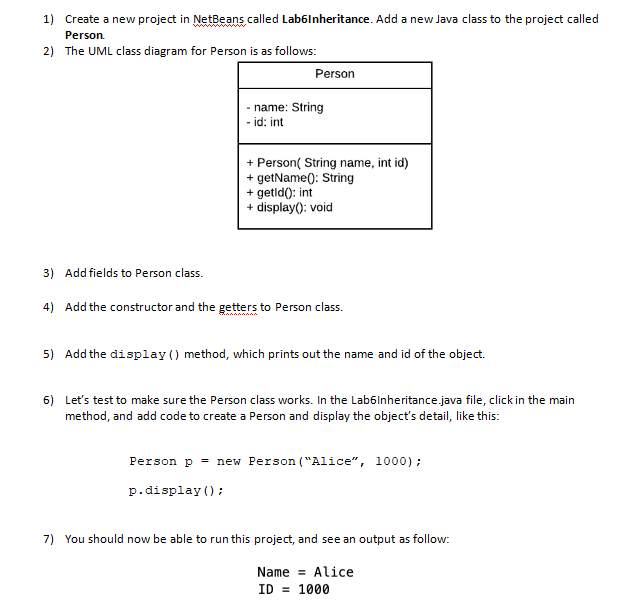I need help for part B and C 1) Create a new project in NetBeans called Lab6Inheritance. Add a new Java class to the project called Person. 2) The UML class diagram for Person is as follows: Person - name: String - id: int + Person( String name, int id) + getName(): String + getido: int + display(): void 3) Add fields to Person class. 4) Add the constructor and the getters to person class. 5) Add the display() method,...

• ### Problem 2 Using the Album and Music StoreDemo from problem 1, write a method to find...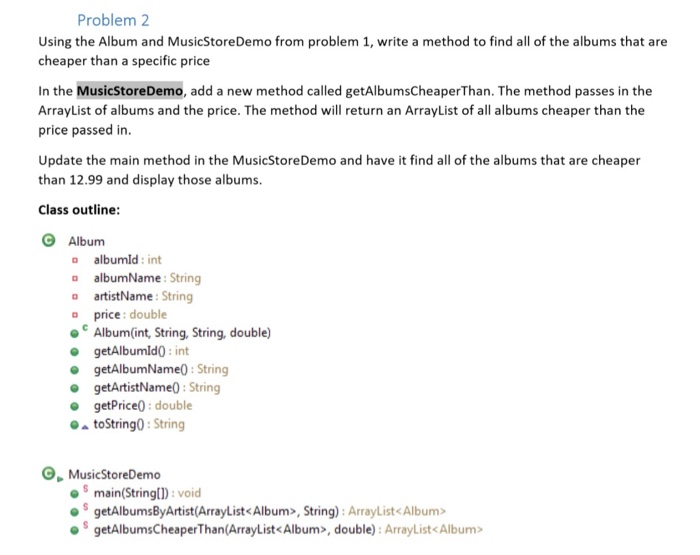Problem 2 Using the Album and Music StoreDemo from problem 1, write a method to find all of the albums that are cheaper than a specific price In the MusicStoreDemo, add a new method called getAlbumsCheaperThan. The method passes in the ArrayList of albums and the price. The method will return an ArrayList of all albums cheaper than the price passed in. Update the main method in the Music Store Demo and have it find all of the albums that...